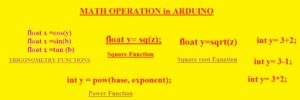Hello, readers welcome to the new post. Today we will learn HOW TO DO MATH OPERATION in ARDUINO. Arduino is a controller that is used to perform different simulations and tasks in engineering projects. Now we will use the Arduino program to perform the different maths operations. We will have a detailed overview of mathematical operators and their uses in Arduino.

We will have a look at different maths operations such as square, square root, power, and trigonometry. So let’s get started

### Different MATHEMATICAL OPERATORS

• The main operators used in maths are plus, + minus *  for multiplication, and / for division. = sign is called the assignment operator which is used to assign the value of a variable.
• Let’s suppose we have integer Y and fix its value equal to two then equation we have
• int y=2;
• It indicates the 2 integers is stored in variable y
• int y= 3+2;
• Through this function there is result 3+2 (five) is stored in variable y
• For subtraction (-) subtraction operator is used
• int y= 3-1;
• The result will be stored in the y variable
• For multiplication * operator is used
• int y= 3*2;
• output six is stored in variable y
• For division/division operator used
• int y=6/2;
• output three will be stored in variable y
• With that variables are also used to perform mathematical operations. Let’s suppose we have two variables c and d instead of whole numbers
• int y= c/d;
• Let us perform division through the use of variables and divide 7 by 3. The code is written here

void setup() {

Serial.begin(9600);

int y = 7/ 3;

Serial.print(x);

}

• The answer will be 2.3 but if we operate the code a number 3 will be printed to the serial monitor. It is due to y being an integer data type. Int command only works for the whole number so 2.3 round off to 2.0
• Let’s suppose that y is float and decimals will add to the whole number
• float y = 7.0 / 3.0;
• The answer will be 2.3### Order of Operations

• Let us suppose that we have an equation having many operators as shown below
• int x=7 +2 * 5-2 /3;
• In this case, we have to follow the order for operations to find the correct answers
• Here is the order mentioned that you must follow to solve the equations
• Parentheses
• Exponents
• Multiplication
• Division
• Subtraction
• first, we have to solve parentheses then exponents after that multiplication and division. Then addition and at last subtraction done

### TRIGONOMETRY FUNCTIONS in Arduino

• Arduino has features to solve the trigonometry equations syntax used for functions of cosine, sine and tangent are given
• float x =cos(y)
• float x =sin(b)
• float x =tan (b)

### Square Function in Arduino

• Arduino is equipped with the function to solve the square of the number
• float y= sq(z);

### Square root Equation in Arduino

• The square root function can be used in Arduino to find the square root of a number
• float y=sqrt(z)

### Power Function in Arduino

• The power function can be used to find the value of the base raised to the power of exponent the syntax for this purpose in Arduino is given here
• int y = pow(base, exponent);
• let’s suppose we have to find the power value of 52 and then put these values in the above equation
• int y= pow(5, 2);

### Maximum and Minimum Functions

• Through the use of Arduino, we can find the maximum and minimum values from pair of numbers
• the min() will be used for a minimum value of two numbers
• int y=min(c, d);
• min() will define the smaller number of two and variables c and d can be any data type
• max() function will find the larger values of these two
• int y =max (c, d);

That is all about the MATH OPERATION in ARDUINO  all operations used in math are also can be used in Arduino to solve different equations. Thanks for reading have nice day